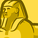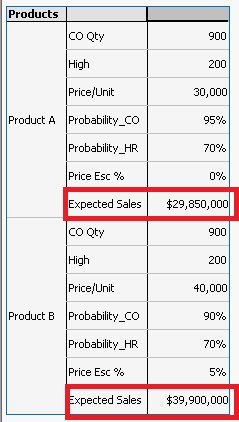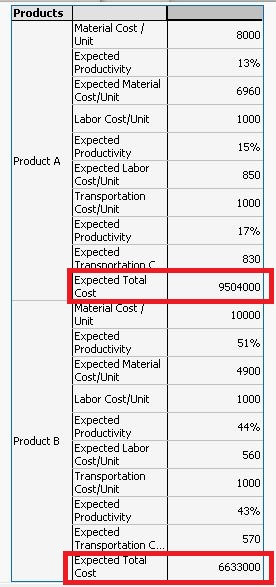# QlikView App Dev

Discussion Board for collaboration related to QlikView App Development.

Announcements
On May 18th at 10AM EDT we will answer your QlikView questions live. REGISTER
cancel
Showing results for
Did you mean:Creator

## How to add sales of two products

Hi,

I have a scenario where i have to add total sales(Expected Sales) of two products(Product A and Product B).I want to get the result as \$69,750,000.

Kindly help.

Please find the Qvw file attached. !

1 Solution

Accepted SolutionsMVP

May be this:

=Sum(TOTAL Aggr((if(Products='Product A' or Products='Product B',[Confirmed Orders(Qty)])

*(if(Products='Product A',30000,40000))

*(1+if(Products='Product A',Price_Esc_A_%,Price_Esc_B_%))

*(if(Products='Product A',Profitability_CO_A,Profitabilty_CO_B)))

+(if(Products='Product A' or Products='Product B',[High Risk(Qty)])

*(if(Products='Product A',30000,40000))

*(1+if(Products='Product A',Price_Esc_A_%,Price_Esc_B_%))

*if(Products='Product A',\$(High_Risk_A),\$(High_Risk_B))), Products))8 RepliesMVP

May be this:

=Sum(TOTAL Aggr((if(Products='Product A' or Products='Product B',[Confirmed Orders(Qty)])

*(if(Products='Product A',30000,40000))

*(1+if(Products='Product A',Price_Esc_A_%,Price_Esc_B_%))

*(if(Products='Product A',Profitability_CO_A,Profitabilty_CO_B)))

+(if(Products='Product A' or Products='Product B',[High Risk(Qty)])

*(if(Products='Product A',30000,40000))

*(1+if(Products='Product A',Price_Esc_A_%,Price_Esc_B_%))

*if(Products='Product A',\$(High_Risk_A),\$(High_Risk_B))), Products))Creator
Author

Thank you so much SunnyMVP

No problem at allCreator
Author

Hi Sunny,

I am facing the same issue with the Expected total cost in the second table. could you please help ,me  to add those values. Please find the snapshot below:I want to add the Expected Total cost of both the products. Kindly help.MVP

You won't be able to use the column labels, but this gets you what you are looking for:

=Sum(TOTAL Aggr((if(Products='Product A' or Products='Product B',if(Products='Product A',[Material Cost / Unit]*(1-Expected_Productivity_1),[Material Cost / Unit]*(1-Expected_Productivity_1_B)))*(sum(if(Products='Product A' or Products='Product B',[Confirmed Orders(Qty)])) + sum(if(Products='Product A' or Products='Product B',[High Risk(Qty)]))))

+(if(Products='Product A' or Products='Product B',if(Products='Product A',[Labor Cost/Unit]*(1-Expected_Productivity_2),[Labor Cost/Unit]*(1-Expected_Productivity_2_B)))*(sum(if(Products='Product A' or Products='Product B',[Confirmed Orders(Qty)])) + sum(if(Products='Product A' or Products='Product B',[High Risk(Qty)]))))

+(if(Products='Product A' or Products='Product B',if(Products='Product A',[Transportation Cost/Unit]*(1-Expected_Productivity_3),[Transportation Cost/Unit]*(1-Expected_Productivity_3_B)))*(sum(if(Products='Product A' or Products='Product B',[Confirmed Orders(Qty)])) + sum(if(Products='Product A' or Products='Product B',[High Risk(Qty)])))), Products))Creator
Author

Thank you so much SunnyCould you please tell how to use those expressions in text box? i have to show the total expected sales in text box but when i use those exp in text box i am getting blank.

Thanks once again for your helpMVP

Seems to be working for me:

1)

=Money(Sum(Aggr((if(Products='Product A' or Products='Product B',[Confirmed Orders(Qty)])

*(if(Products='Product A',30000,40000))

*(1+if(Products='Product A',Price_Esc_A_%,Price_Esc_B_%))

*(if(Products='Product A',Profitability_CO_A,Profitabilty_CO_B)))

+(if(Products='Product A' or Products='Product B',[High Risk(Qty)])

*(if(Products='Product A',30000,40000))

*(1+if(Products='Product A',Price_Esc_A_%,Price_Esc_B_%))

*if(Products='Product A',\$(High_Risk_A),\$(High_Risk_B))), Products)))

2)

=Money(Sum(Aggr((if(Products='Product A' or Products='Product B',if(Products='Product A',[Material Cost / Unit]*(1-Expected_Productivity_1),[Material Cost / Unit]*(1-Expected_Productivity_1_B)))*(sum(if(Products='Product A' or Products='Product B',[Confirmed Orders(Qty)])) + sum(if(Products='Product A' or Products='Product B',[High Risk(Qty)]))))

+(if(Products='Product A' or Products='Product B',if(Products='Product A',[Labor Cost/Unit]*(1-Expected_Productivity_2),[Labor Cost/Unit]*(1-Expected_Productivity_2_B)))*(sum(if(Products='Product A' or Products='Product B',[Confirmed Orders(Qty)])) + sum(if(Products='Product A' or Products='Product B',[High Risk(Qty)]))))

+(if(Products='Product A' or Products='Product B',if(Products='Product A',[Transportation Cost/Unit]*(1-Expected_Productivity_3),[Transportation Cost/Unit]*(1-Expected_Productivity_3_B)))*(sum(if(Products='Product A' or Products='Product B',[Confirmed Orders(Qty)])) + sum(if(Products='Product A' or Products='Product B',[High Risk(Qty)])))), Products)))Creator
Author

Hey Sunny you are genius. Appreciate your knowledge and your great helpThank you so much!!